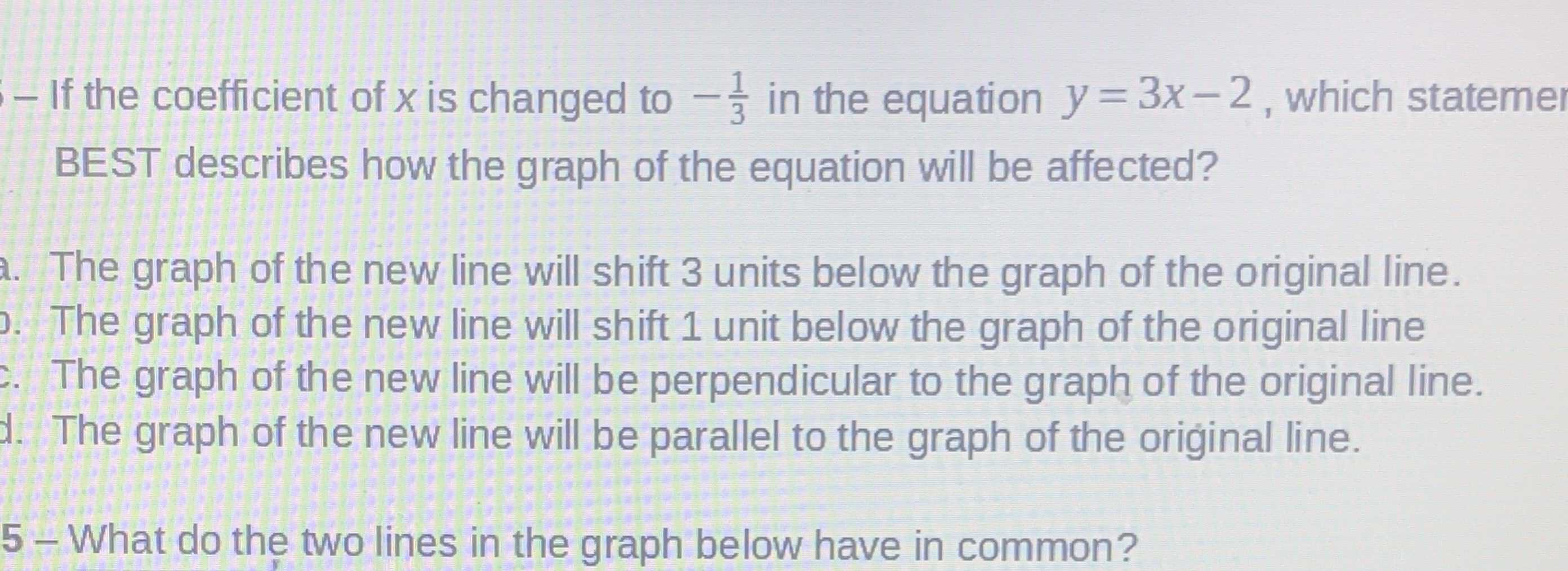### ¿Todavía tienes preguntas de matemáticas?

Pregunte a nuestros tutores expertos
Algebra
Pregunta- If the coefficient of $$x$$ is changed to $$- \frac { 1 } { 3 }$$ in the equation $$y = 3 x - 2 ,$$ which statemer BEST describes how the graph of the equation will be affected?

A. The graph of the new line will shift $$3$$ units below the graph of the original line. 6.

B. The graph of the new line will shift $$1$$ unit below the graph of the original line

C. The graph of the new line will be perpendicular to the graph of the original line.

D. The graph of the new line will be parallel to the graph of the oriḡinal line.